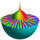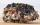# Roman numerals 2+

Add up the number written in Roman numerals. Write the results as roman numbers.

Result

DCCLXXXVIII + DCCLXVII = (Correct answer is: MDLV)CCXXII + CMLXVIII = (Correct answer is: MCXC)DXXXIV + DCCCLXV = (Correct answer is: MCCCXCIX)CCCLXXXIV + CMXCVII = (Correct answer is: MCCCLXXXI)DCCXLVII + CCLXII = (Correct answer is: MIX)DVIII + CMXXVIII = (Correct answer is: MCDXXXVI)DCCCLXXX + DCCLXXXVII = (Correct answer is: MDCLXVII)CDXXVIII + DCXCII = (Correct answer is: MCXX)CCCLXXV + DCCXCI = (Correct answer is: MCLXVI)CDXXXVIII + CXXXVIII = (Correct answer is: DLXXVI)### Step-by-step explanation:

DCCLXXXVIII + DCCLXVII = 788 + 767 = 1555 = MDLV
CCXXII + CMLXVIII = 222 + 968 = 1190 = MCXC
DXXXIV + DCCCLXV = 534 + 865 = 1399 = MCCCXCIX
CCCLXXXIV + CMXCVII = 384 + 997 = 1381 = MCCCLXXXI
DCCXLVII + CCLXII = 747 + 262 = 1009 = MIX
DVIII + CMXXVIII = 508 + 928 = 1436 = MCDXXXVI
DCCCLXXX + DCCLXXXVII = 880 + 787 = 1667 = MDCLXVII
CDXXVIII + DCXCII = 428 + 692 = 1120 = MCXX
CCCLXXV + DCCXCI = 375 + 791 = 1166 = MCLXVI
CDXXXVIII + CXXXVIII = 438 + 138 = 576 = DLXXVIDid you find an error or inaccuracy? Feel free to write us. Thank you!## Related math problems and questions:

• Roman numerals +Add up the number written in Roman numerals. Write the results as a decimal number.
• Roman numerals 2-Subtract up the number written in Roman numerals. Write the results as Roman numbers.
• Roman numeralsWrite numbers written in Roman numerals as decimal.Added together and write as decimal number: LXVII + MLXIVAdd and write the result again as hours, minutes, seconds: 2hodiny45min15s + 1h20m50s =Add two mixed fractions: 2 4/6 + 1 3/6
• Mistake in expressionWhile attempting to multiply the expression (2 - 5i)(5 + 2i) a student made a mistake. (2 - 5i)(5 + 2i) = 10 + 4i - 25i - 10i2 = 10 + 4(-1) - 25(-1) - 10(1) = 10 - 4 + 25 - 10 = 21 Complete the explanation and correct the error. Hint: The student inc
• Written numberPlace+values x ten thousands =30 thousands
• DozenWhat is the product of 26 and 5? Write the answer in an Arabic numeral. Add up the digits. How many of this is in a dozen? Divide #114 by this
• David numberJana and David train the addition of the decimal numbers so that each of them will write a single number and these two numbers then add up. The last example was 11.11. David's number had the same number of digits before the decimal point, the Jane's numbeAdd marks (+, -, *, /, brackets) to fullfill equations 1 3 6 5 = 10 This is for the 4th grade of the primary school - with no negative numbers yetAdd this two mixed numbers: 1 5/6 + 2 2/11=Roman is ranked 12th highest and eleventh lowest pupil. How many classmates does Roman have?Nicol mistake when calculate in school. Instead of add number 20 subtract it. What is the difference between the result and the right result?Write the smallest and largest 2-digit natural number.Number 5040 divide by the number 5 and by number 10: a = 5040: 5 b = 5040: 10On the 4-th stop take on 56 and take off 38 passengers. How many were added (write as positive number) or shrunk (write as negative number) the count of passengers?